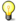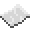# math.tanNote: The argument must be given in radians. If it is in degrees, use the math.rad function to convert it.ExamplePrint the tangent of user input
Code
```<nowiki>
</nowiki>
```
Output Depends. If the user wrote `45`, the output would be `1`.

math.tan
Function
Syntax
math.tan(
• num : number
)

Returns number
API math
Source Lua (source)

Returns the tangent of num.